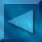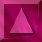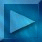Previous : Calculus of the Likelihood  Next : Sampling the densities Notations

 Log-Likelihood derivatives

We have to minimize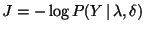w.r.t.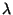and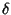.

Therefore, let us calculate its derivatives w.r.t. the hyperparameters :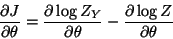we get :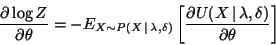where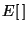= expectation calculated with  X samples
which are images following the probability law :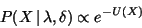.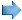We must also calculate two types of expectations :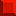a priori law: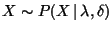to take into account the constraintsa posteriori law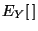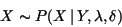w.r.t. the data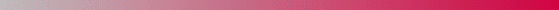André Jalobeanu - 24 Aug 1998